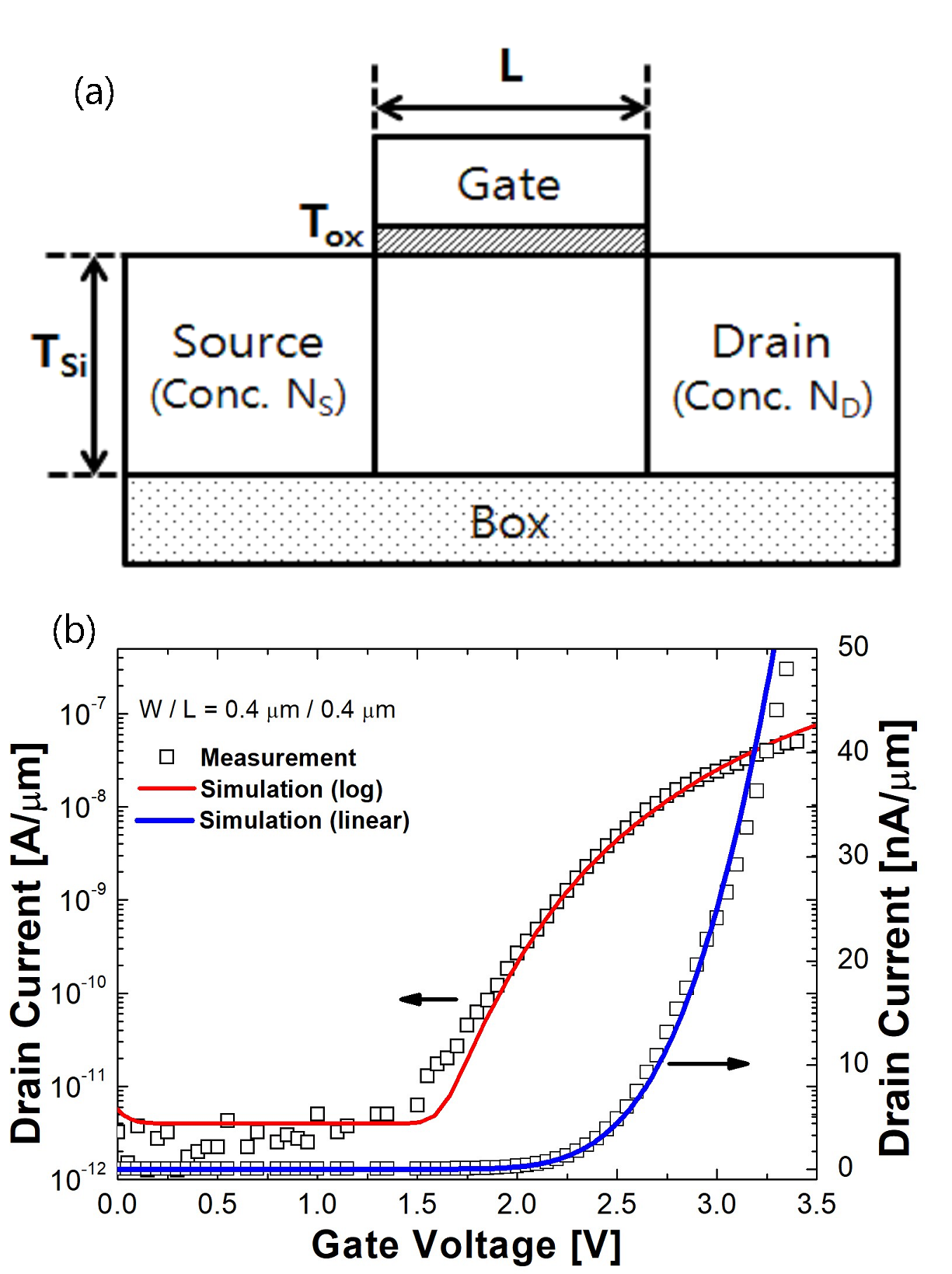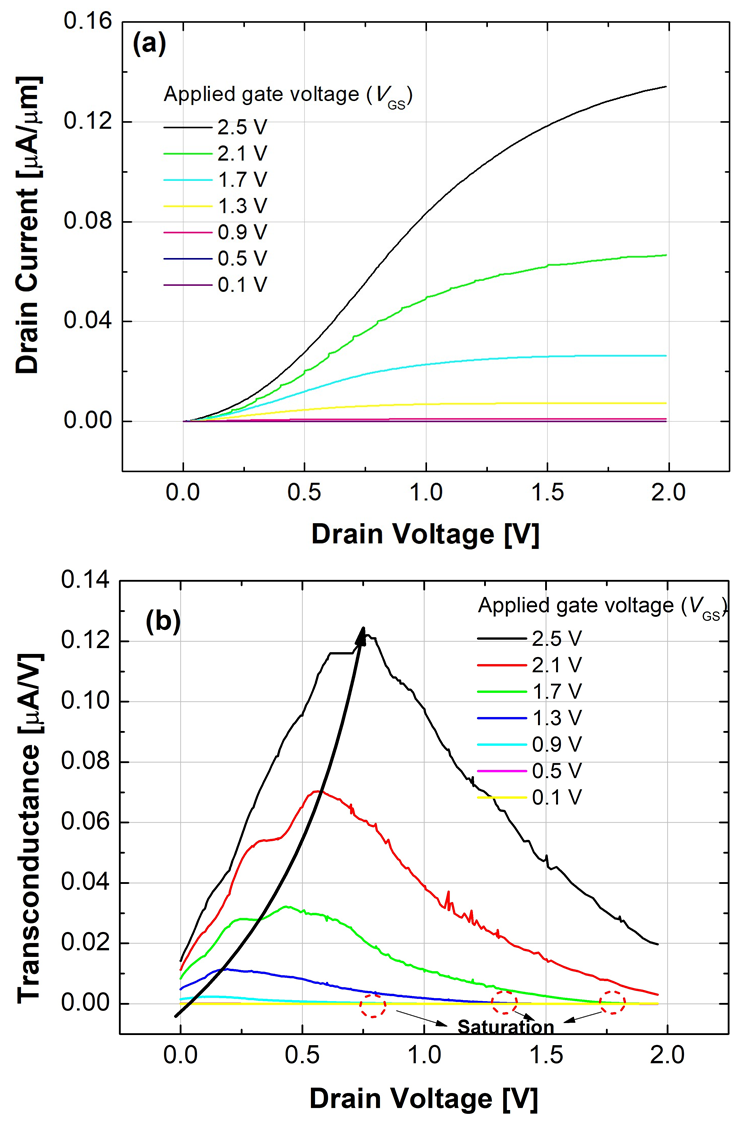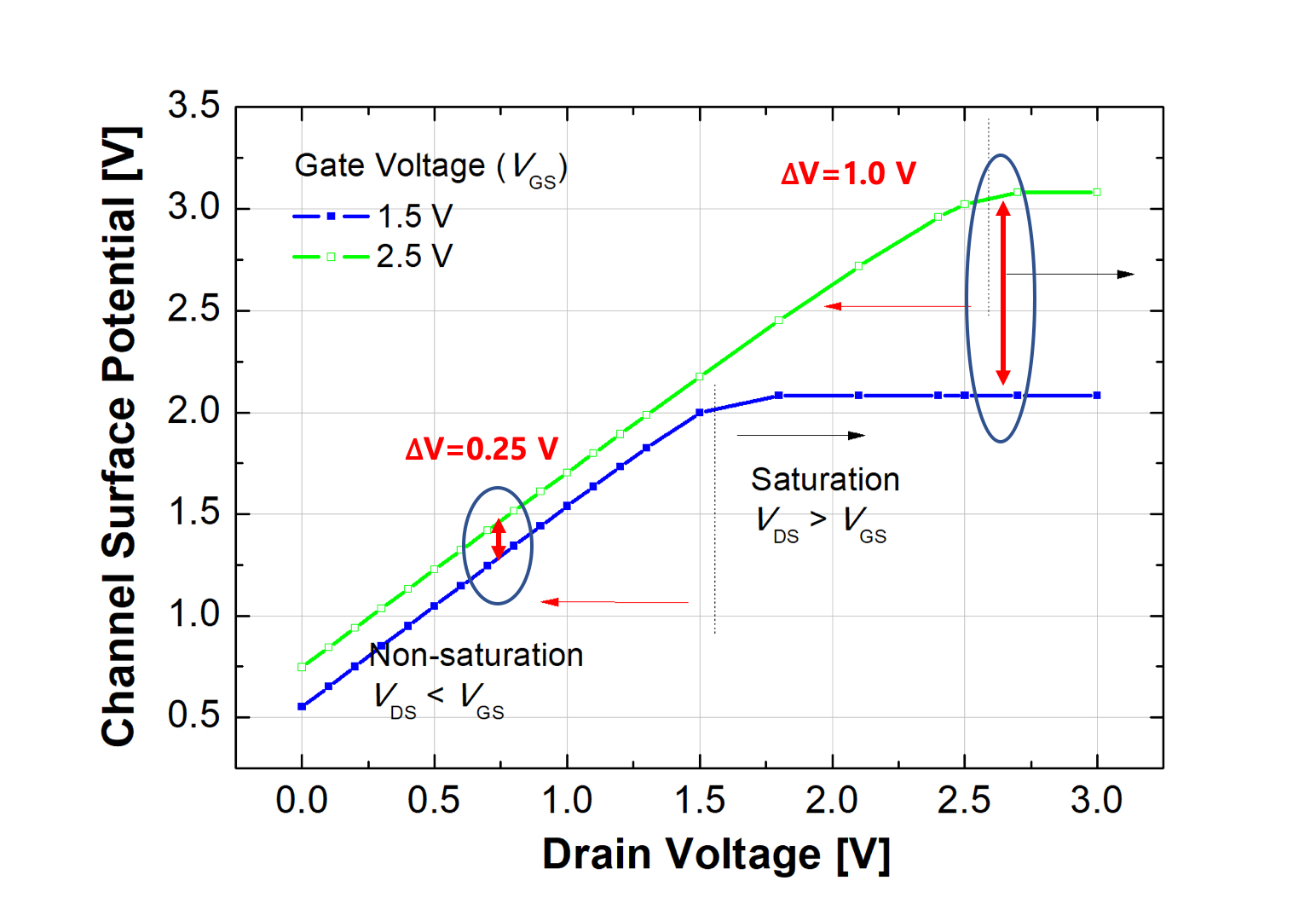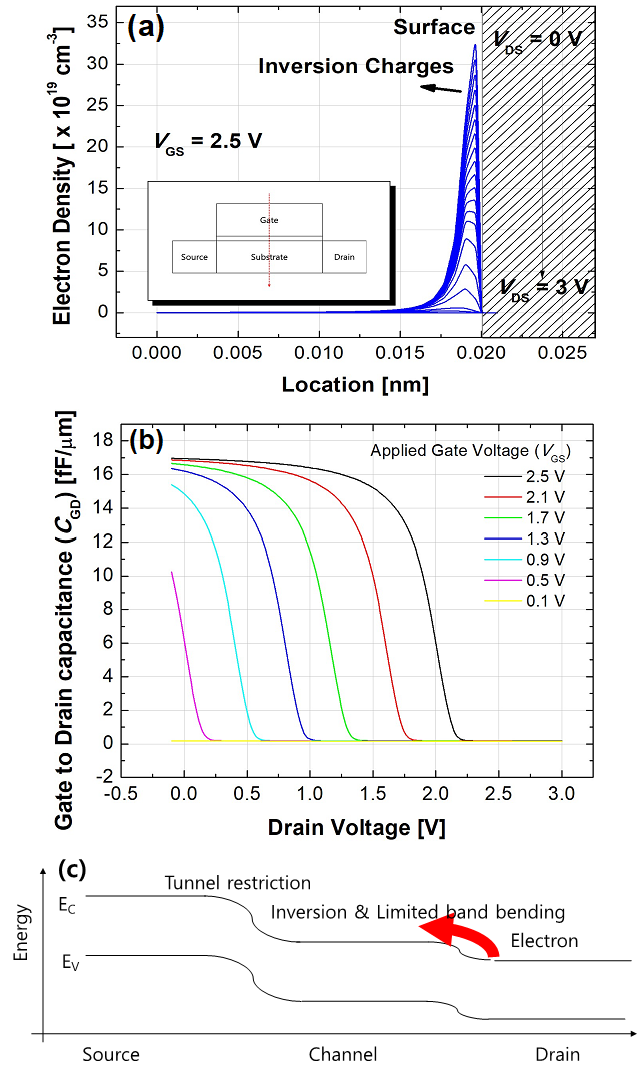Mobile QR Code1. (School of Electrical Engineering, Pukyong National University, 45 Yongso-ro, Nam-gu, Busan 48513, Korea)
2. (Department of Electrical and Computer Engineering, Ajou University, Suwon 16499, Korea)

Tunnel field-effect transistor, TFET, nonlinear output characteristics, BTBT

## I. INTRODUCTION

Recently, tunnel field-effect transistor (TFET) has been researched widely due to their applicability for the low-power operations (1-4). In case of metal-oxide-semiconductor FET (MOSFET), which is conventionally utilized in the various circuits, there exists theoretical limit of 60 mV/dec subthreshold swing (SS) at the room temperature (RT) because its carrier injection is based on the thermionic emission (5,6). On the other hand, TFET is relatively independent to the Boltzmann distribution since the function tail is removed by forbidden gap. In addition, its dominant carrier injection mechanism is a band-to-band tunneling (BTBT) (7,8). Thus, the SS can be reduced to less than Boltzmann limit (i.e., 60 mV/dec at RT), which allows the supply voltage ($V_{DD}$) to be scaled down drastically maintaining high ON-state current ($I_{ON}$). However, TFET still has unsolved nonlinear current (NLC) problem in output characteristics especially at low drain bias ($V_{DS}$) (9,10). The NLC issue (i.e., small output conductance) significantly degrades the performance of TFET circuits such as rising and falling delay. However, the analysis of NLC have not been studied rigorously, yet.

This paper aims to study a fundamental origin of the NLC in output characteristics through technology computer-aided design (TCAD) simulations. Especially, the analysis of NLC is mainly performed to identify channel potential pinning in particular bias condition. The details about this study are as follow. After briefly explain the device process flow, BTBT model in the simulation tool is calibrated according to the parameters from the fabricated devices. Then, NLC phenomena in output characteristics are examined with the help of the energy band diagrams and channel potential. In addition, gate-to-drain capacitance ($C_{GD}$) and charge density in channel are also discussed.

## II. DEVICE AND SIMULATION

First of all, before the TCAD simulations, a BTBT model calibration is performed based on experimental results. Fig. 1 shows the device structure of TFET. It is fabricated on a (100) $p$-type silicon-on-insulator (SOI) wafer. First, SOI thickness is thinned by using wet oxidation and wet etching processes. Active region is defined using photolithography and reactive ion etching (RIE) process. After that, gate oxide ($T_{OX}$ = 3 nm) is grown by dry oxidation. An n-type doped polycrystalline-Si gate is deposited by using low pressure chemical vapor deposition (LPCVD). After gate patterning, asymmetric source and drain (S/D) are formed through photolithography and ion implantation processes. In detail, BF$_{2}$ with 8×1014 cm$^{-2}$-dose, 7º tilt, and 10 keV-acceleration energy is used for source implantation while drain implantation is performed by As ions with the same condition. The dopant activation is implemented by rapid thermal process (RTP) with 900 ºC and 5 sec. Finally, as a back-end-of-line (BEOL) processes, a tetra-ethyl-ortho-silicate (TEOS) is deposited by plasma-enhanced CVD (PECVD) as an inter-layer dielectric (ILD) and metal layers (Ti/TiN/Al/TiN stacks) are deposited by physical vapor deposition (PVD) after contact hole formation. All the transfer characteristics are measured at $V_{DS}$ of 0.1 V and TCAD simulations are performed by using Synopsys SentaurusTM (11). A BTBT generation rate per unit volume ($G$) defined as

##### (1)
$$G=A\left(\frac{F}{F_{0}}\right)^{P} \exp \left(-\frac{B}{F}\right)$$

in the uniform electric field limit where $F_{0}$ = 1 V/m and $P$ = 2.5 for indirect tunneling (11). The prefactor ($A$) and the exponential factor ($B$) are Kane parameters while the F is electric field. The $A$ and $B$ values are chosen for the best fitting between the simulated and measured data (Fig. 1(b)) (12,13). From the results, it is found that both linear and log scales of the transfer characteristics are well matched. The extracted $A$ and $B$ parameters of the BTBT model in Si TFET are 4×10$^{14}$ cm$^{-1}$s$^{-1}$ and 9.9×10$^{6}$ V/cm, respectively.

Fig. 1. Cross-sectional views of TFET for BTBT calibration, (b) Calibrated transfer characteristics from simulation and measurement results for TFET.Table 1. Simulation parameters

 Abbreviations Description Quantity $T_{Si}$ Body thickness 100 nm $T_{OX}$ Gate dielectric thickness 1 nm $L$ Channel length 500 nm $W$ Channel width 1 mm $N_{D}$ Drain doping concentration n-type 1020 cm-3 $N_{B}$ Body doping concentration p-type 1015 cm-3 $N_{S}$ Source doping concentration p-type 1020 cm-3

The simulated TFET for NLC analysis has same structure in Fig. 1(a) and it is designed to analyze output characteristics with the low $V_{DS}$, which is known as linear region. The simulated TFET features 100 nm-thick body thickness ($T_{Si}$), 1 nm-$T_{OX}$ and 500 nm channel length ($L$) to suppress short channel effects. In addition, an abrupt S/D doping profiles are assumed to minimize depletion region between S/D and channel. The specific information are summarized in Table 1. A dynamic nonlocal BTBT and a Shockley–Read–Hall generation-recombination models are used for the simulation (14).

## III. RESULTS AND DISCUSSION

The output characteristics of the TFET are studied by increasing gate voltage ($V_{GS}$) from 0.1 to 2.5 V with 0.4 V step (Fig. 2(a)). The output current can be classified into two characteristics. First, the drain current ($I_{D}$) cannot be increased regardless of $V_{GS}$ at a very low $V_{DS}$. In this operating condition, the $I_{D}$ is increased exponentially as a function of $V_{DS}$. Second, as the $V_{DS}$ increases, the increasing rate of $I_{D}$ gradually reduced and finally the $I_{D}$ is saturated. The NLC is confirmed at the first characteristic. The nonlinear characteristic severely degrades $I_{D}$ compared with linearly increasing case (e.g., MOSFET). The low current at low $V_{DS}$ is confirmed by transconductance curves in Fig. 2(b). Transconductance shows very small values near zero $V_{DS}$. The small transconductance significantly degrades the performance of TFET circuits such as propagation delay due to the low current drivability.

Fig. 2. (a) Output characteristics of TFET with various $V_{GS}$, (b) Transconductance as a function of $V_{DS}$ with the various $V_{GS}$.Fig. 3. Extracted channel potential as a function of $V_{DS}$ with the various $V_{GS}$. The channel potential is extracted at 1 nm below the gate oxide according to the source-to-drain direction.It is well known that the current in TFET is determined by the BTBT rate at source-to-channel junction. Therefore, in order to analyze NLC behavior, a channel potential is extracted as a function of $V_{DS}$ with 1.5 V and 2.5 V of $V_{GS}$ (Fig. 3). The results show that the channel potential can be classified into two regions. First, the $V_{DS}$ < $V_{GS}$ conditions are defined as non-saturation regions. Note that, the NLC is confirmed at this region. In this region, there is only 0.25 V-potential difference between two curves even though the increased amount of $V_{GS}$ is 1 V (i.e., from 1.5 V to 2.5 V). In other words, the channel potential is rarely modulated by the $V_{GS}$ and it is concluded that the $I_{D}$ is restricted by the pinned channel potential. Second, the $V_{DS}$ > $V_{GS}$ conditions are defined as saturation regions, in which the channel potential remains a constant although the $V_{DS}$ is increased. In addition, the saturation regions in terms of $V_{GS}$ could be also confirmed in Fig. 2(b), where the transconductance is zero. The difference of channel potential between two curves is about 1.0 V, corresponded to the $V_{GS}$ difference. It means that the channel potential is modulated proportionally to the applied $V_{GS}$ in this region.

Fig. 4. (a) Electron density distributions at the channel as the $V_{DS}$ increases. They are extracted along the depth direction as shown in the inset, (b) Extracted $C_{GD}$ as a function of $V_{DS}$ with the various $V_{GS}$. As the $V_{GS}$ increases, the $C_{GD}$ is shifted to the positive direction, (c) Illustration of energy band diagram with channel inversion.In order to confirm the cause of the channel potential pinning in non-saturation region rigorously, the density of the electron charges (inversion charges) on channel is investigated (Fig. 4(a)). The results show that the electrons are piled on the channel at the 2.5 V of $V_{GS}$ and 0 V of $V_{DS}$. In addition, with increasing $V_{DS}$, the electrons in the channel are decreased. It means that the modulating channel potential is restricted by the inversion charges from drain. Then, to investigate the amount of inversion changes depending on the bias condition, the $C_{GD}$ is simulated with various $V_{GS}$ (Fig. 4(b)). It is well corresponded to the results shown in Fig. 4(a) that the $C_{GD}$ is decreased as the $V_{DS}$ is increased. In addition, when higher $V_{GS}$ is applied, $C_{GD}$ is decreased at more positive $V_{DS}$. The reason is that with low $V_{DS}$ or high $V_{GS}$, there is low band bending in the channel-to-drain junction, which implies a small energy barrier and high inversion charges in the channel (Fig. 4(c)) (14-16). Therefore, when a low $V_{DS}$ is applied, the channel potential is rarely modulated by $V_{GS}$ and limits the tunneling from the source to the channel, results in the NLC phenomenon.

## IV. CONCLUSIONS

This paper aim to study the NLC behavior in output characteristics which have been detected in TFET operation. When low $V_{DS}$ is applied in TFET, it has been revealed that inversion carriers of TFETs are provided from the drain. The inversion charges result in inhibiting channel band bending, which is modulated by $V_{GS}$. Thus, the dominant NLC mechanism is found that the inversion charges from drain region confined the channel modulation.

### ACKNOWLEDGMENTS

This research was supported in part by the Brain Korea 21 Plus Project, in part by the MOTIE/KSRC under Grant 10080575 (Future Semiconductor Device Technology Development Program), and in part by the NRF of Korea funded by the MSIT under Grant NRF-2019M3F3 A1A03079739 and NRF-2019M3F3A1A02072091 (Intelligent Semiconductor Technology Development Program). The EDA tool was supported by the IC Design Education Center (IDEC), Korea.

### REFERENCES

1
Choi W. Y., Park B.-G., Lee J. D., Liu T.-J. K., Aug 2007, Tunneling field-effect transistors (TFETs) with subthreshold swing (SS) less than 60 mV/dec, IEEE Electron Device Letters, doi: 10.1109/LED.2007.901273, Vol. 28, No. 8, pp. 743-7452
Wang P.-Y., Tsui B.-Y., Jan 2016, Band engineering to improve average subthreshold swing by suppressing low electric field band-to-band tunneling with epitaxial tunnel layer tunnel FET structure, IEEE Transactions on Nanotechnology, doi: 10.1109/TNANO.2015.2501829, Vol. 15, No. 1, pp. 74-793
Kim S. W., Choi W. Y., Kim H., Sun M. C., Kim H. W., Park B.-G., 2012, Investigation on hump effects of L-shaped tunneling filed-effect transistors, IEEE Silicon Nanoelectronics Workshop (SNW), Honolulu, HI, 2012, doi: 10.1109/SNW.2012.6243306, pp. 1-24
Mishra A., Pattanaik M., Sharma V., 2013, Double gate vertical tunnel FET for hybrid CMOS-TFET based low standby power logic circuits, Annual International Conference on Emerging Research Areas and International Conference on Microelectronics, Communications and Renewable Energy, Kanjirapally, doi: 10.1109/AICERA- ICMiCR.2013.6575992, pp. 1-45
Knoch J., 2016, Chapter Eight - nanowire tunneling field-effect transistors, Semiconductors and Semimetals, http://dx.doi.org/10.1016/bs.semsem.2015.09.005, Vol. 94, pp. 273-2956
Long P., Wilson E., Huang J. Z., Klimeck G., Rodwell M. J. W., Povolotskyi M., Jan 2016, Design and simulation of GaSb/InAs 2D transmission enhanced tunneling FETs, IEEE Electron Device Lett., doi: 10.1109/LED.2015.2497666, Vol. 37, No. 1, pp. 107-1107
Dash S., Sahoo G. S., Mishra G. P., Mar 2016, Subthreshold swing minimization of cylindrical tunnel FET using binary metal alloy gate, Superlattices and Microstructures, https://doi.org/10.1016/j.spmi.2016.01.010, Vol. 91, pp. 105-1118
Usha C., Vimala P., 2015, A tunneling FET exploiting in various structures and different models: A review, International Conference on Innova- tions in Information, Embedded and Communication Systems (ICIIECS), Coimbatore, doi: 10.1109/ICIIECS.2015.7192878, pp. 1-69
Dagtekin N., Mihai Ionescu A., May 2015, Impact of Super-Linear Onset, Off-Region Due to Uni-Directional Conductance and Dominant CGD on Performance of TFET-Based Circuits, IEEE Journal of the Electron Devices Society, doi: 10.1109/JEDS.2014. 2377576, Vol. 3, No. 3, pp. 233-23910
Zhong Y., Chen C., Huang Q., Wang Z., Ye L., Lou J., Huang R., 2018, Current Degradation and Delay Analysis of Series-Connected Circuits Based on Novel TFET Design, IEEE International Conference on Solid-State and Integrated Circuit Technology (ICSICT), Qingdao, doi: 10.1109/ICSICT.2018.8565752, pp. 1-311
Sentaurus Device User Guide, ver. G-2012.06, Synopsys Inc.12
Kane E. O., 1961, Theory of Tunneling, Applied physics lett., doi: 10.1063/1.1735965, Vol. 32, No. 1, pp. 83-9113
Biswas A., Dan S. S., Royer C. L., Grabinski W., Ionescu A. M., 2012, TCAD simulation of SOI TFETs and calibration of non-local band-to-band tunneling model, Microelectronic Engineering, doi: 10.1016/j.mee.2012.07.077, Vol. 98, pp. 334-33714
Lee W., Choi W., Sept 2011, Influence of Inversion Layer on Tunneling Field-Effect Transistors, IEEE Electron Device Lett., doi: 10.1109/LED.2011.2159257, Vol. 32, No. 9, pp. 1191-119315
Safa S., Noor S. L., Khan M. Z. R., 2016, Simulation-based study on the effect of inversion charge layer on triple material double gate Tunnel FET, International Conference on Electrical and Computer Engineering (ICECE), Dhaka, doi: 10.1109/ICECE.2016.7853923, pp. 329-33216
Vishnoi R., Kumar M. J., Feb 2015, An Accurate Compact Analytical Model for the Drain Current of a TFET From Subthreshold to Strong Inversion, IEEE Transactions on Electron Devices, doi: 10.1109/TED. 2014.2381560, Vol. 62, No. 2, pp. 478-484## Author

was born in Seoul, South Korea, in 1985. He received BS. Degrees from Korea Advanced Institute of Science (KAIST), Daejeon, South Korea, in 2009, and M.S. and Ph.D. degrees in electrical engineering from Seoul National University, Seoul, in 2011 and 2016, respectively.

From 2016 to 2020, he joined SK Hynix inc. He is currently an Assistant Professor with the School of Electrical Engineering, Pukyong National University.

was born in Daegu, South Korea, in 1983. He received the B.S., M.S., and the Ph.D. degrees in Electrical Engineering from Seoul National University, Seoul, South Korea, in 2006, 2008, and 2014, respectively.

He had been a post-doctoral scholar at the Department of Electrical Engineering and Computer Sciences, University of California, Berkeley, USA, from 2014 to 2017.

Since 2017, he has been a Faculty Member with Ajou University, Suwon, South Korea, where he is currently an Associate Professor with the Department of Electrical and Computer Engineering.